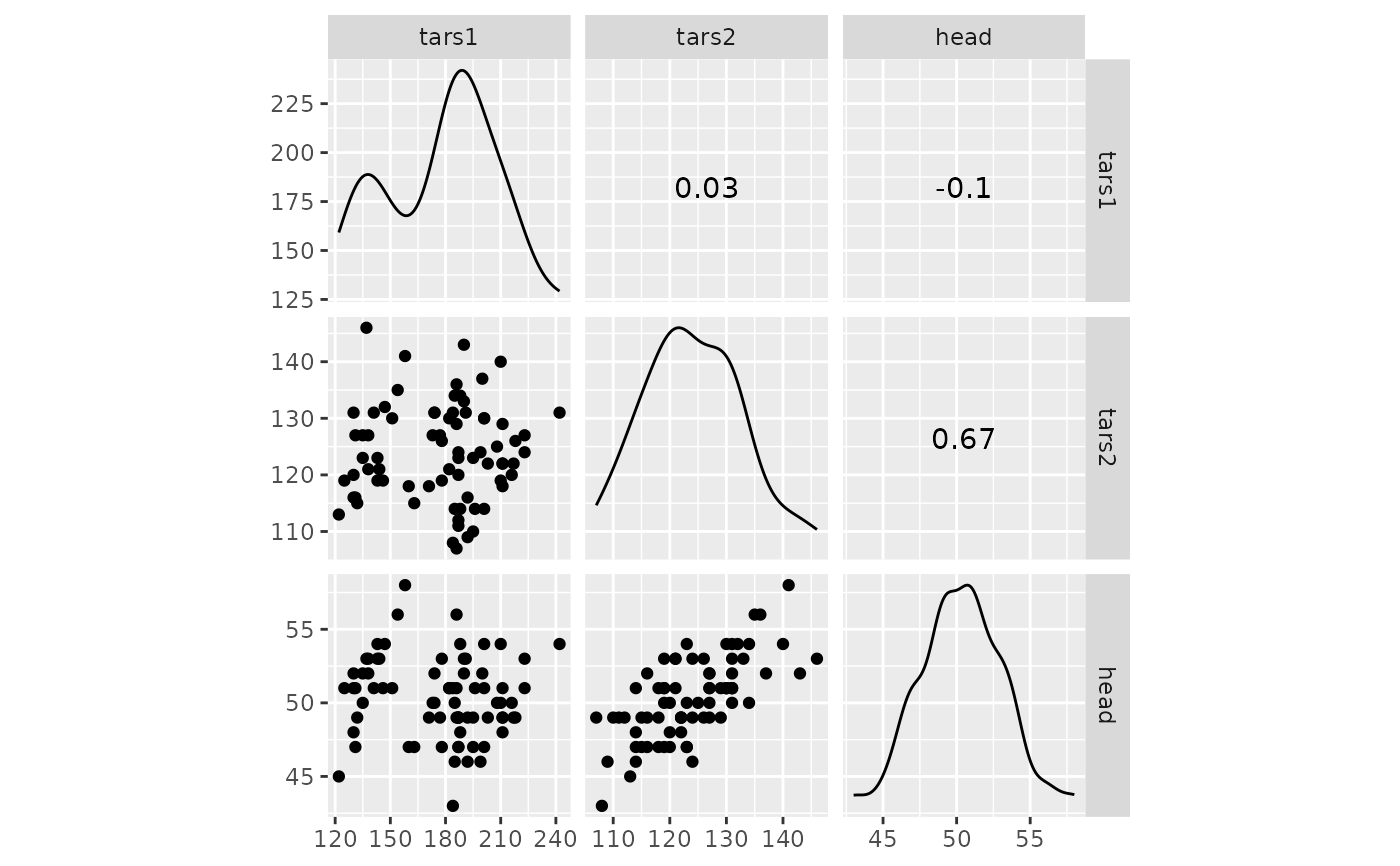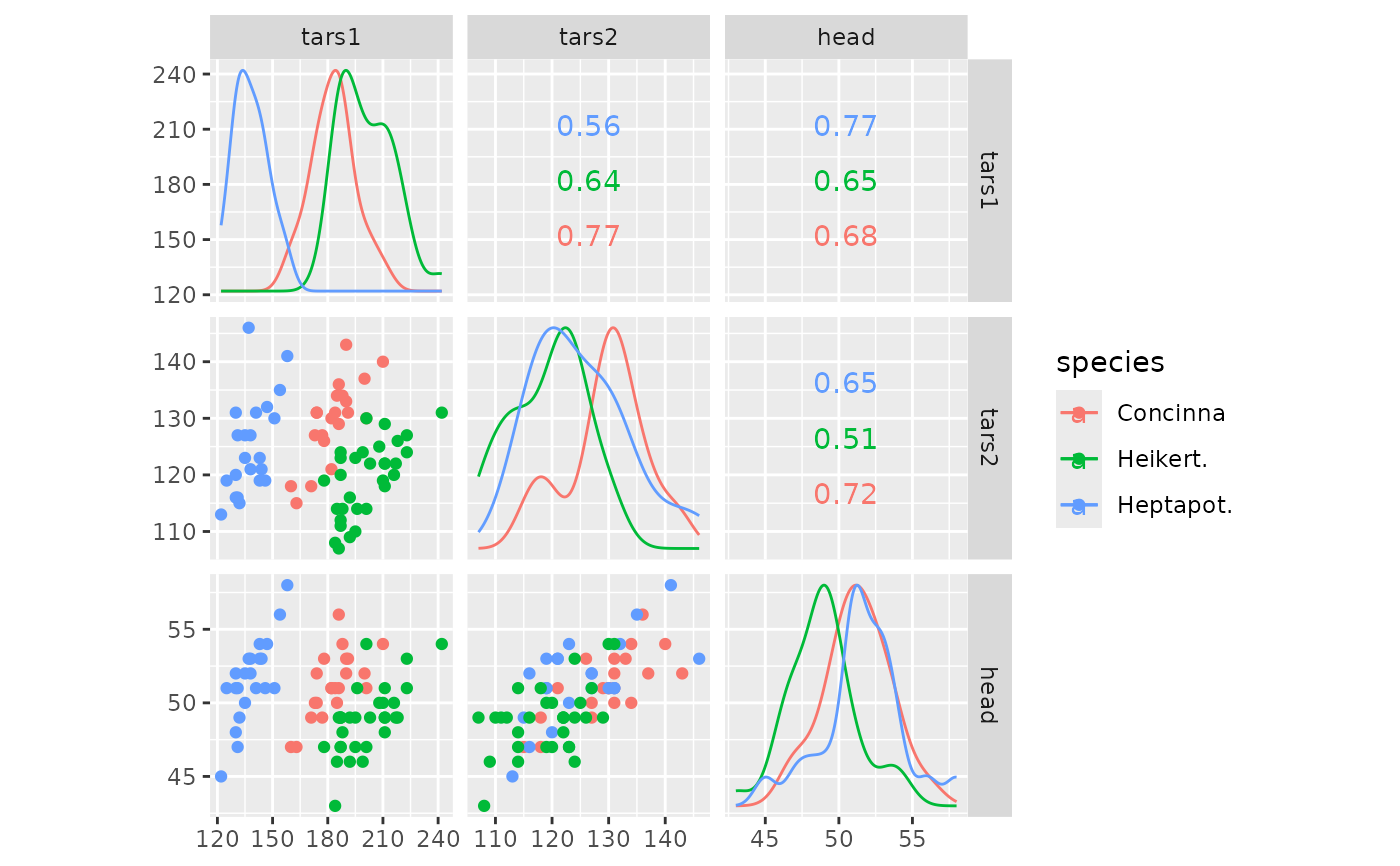This function makes a scatterplot matrix for quantitative variables with density plots on the diagonal and correlation printed in the upper triangle.

ggscatmat(
data,
columns = 1:ncol(data),
color = NULL,
alpha = 1,
corMethod = "pearson"
)

## Arguments

data a data matrix. Should contain numerical (continuous) data. an option to choose the column to be used in the raw dataset. Defaults to 1:ncol(data). an option to group the dataset by the factor variable and color them by different colors. Defaults to NULL, i.e. no coloring. If supplied, it will be converted to a factor. an option to set the transparency in scatterplots for large data. Defaults to 1. method argument supplied to cor

## Author

Mengjia Ni, Di Cook

## Examples

# small function to display plots only if it's interactive
p_ <- GGally::print_if_interactive

data(flea)

p_(ggscatmat(flea, columns = 2:4))p_(ggscatmat(flea, columns = 2:4, color = "species"))